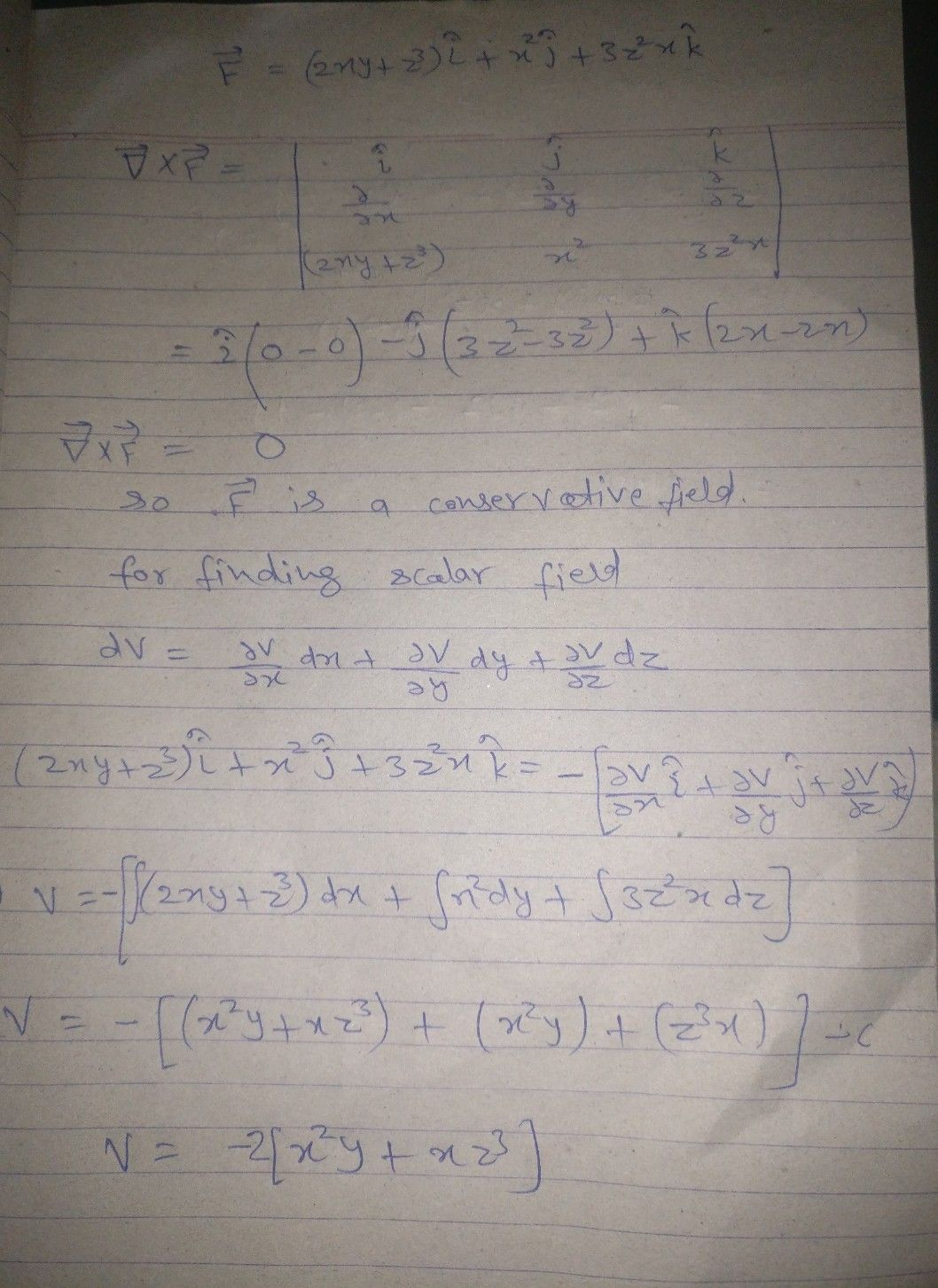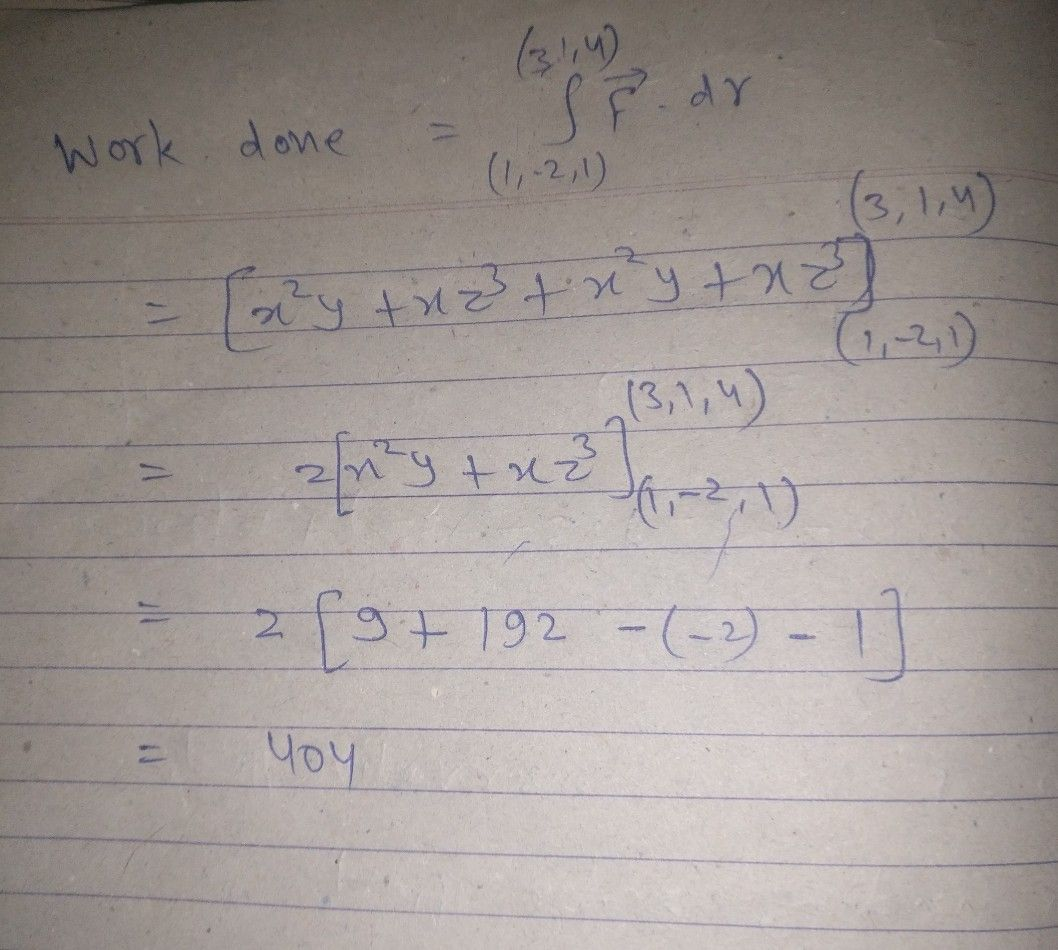Symbol
Problem1. Show that $F=\left(2xy+\bar{z^{3}\right)i+x^{2}+3z^{2}x} k$ is $a$ conservative field. Find its $c230$ potential and also the work done in moving a particle from $\left(1$ $-2,1\right)$ $10\left(3,1,4\right)$
Geometry
Search count: 119
SolutionQanda teacher - kantianil